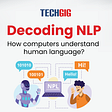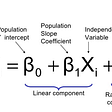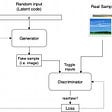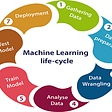# Ensemble Learning

Ensemble learning techniques combine individual model together to improve the stability and predictive power of model. This technique permits higher predictive performance and it combine multiple machine learning models into one predictive model.

Definition :

Certain models do well in modelling on aspect the data., while others do well in modelling another. It takes several simple model and combine, learns on it and it’s outputs to produce the final decision.

The combine strength of the models offsets individual model variance and biased. This provides a composite prediction where final accuracy is better than accuracy of individual model.

# Ensemble method is used for two methods :

1. Sequential ensemble method

- In this base learner are generally consecutively. Basic motivation is use the dependence between the base learners.

- The overall performance of a model can be boosted.

2. Parallel ensemble methods

- In parallel ensemble applied wherever the base learner are generated in parallel. Basic motivation is use independence between the base learner.

# Significance :

Ensemble model is the application of the multiple models to obtain better performance than from single model.

• Robustness – Ensemble model incorporates the predictions from all the base learner.
• - Accuracy – Ensemble models deliver accurate predictions and have improved performance.

# Ensemble Learning Method :

Ensemble Learning creates an ensemble of all well-choose strong and diverse models and we use ‘ Averaging ‘ to find ensemble prediction .

# Bagging / Bootstrap Aggregating (reduce variance)

Bagging or Bootstrap Aggregating “reduces variance” of the estimate by taking the mean of multiple estimate.

# Bagging Algorithm Steps

Create random sampled dataset of the original training data. (Bootstrapping)

Build and fit several. classifier to each of theses diverse copies.

Takes the average of all the predictions to make a final overall prediction.

# Random Forests

Random forest is a good example of ensemble learning in methods :

Random Forest technique combines various decisions tree to produce a more generalised method.

Random Forest are utilised to produce de-correlation decision tree.

Random forest creates random subsets of the features.

Smaller tree are build using these. subsets, creating tree diversity.

# Boosting (reduces bias)

Boosting reduces bias by training weak learners sequentially each trying to correct its predecessor.

# Boosting Algorithm Steps

Train a classifier A1 that best classify the data with respect to accuracy.

Identify the regions where A1 produces error, add weight to them and produce a A2 classifier.

Aggregate those samples for where ‘A1’ gives the different result from ‘A2’ and produce ‘A3’ classifier. Repeat step 2 for a new classifier.

Boosting is technique of changing weak learner into strong learner . Each. new tree is a fit on modified version of original dataset .

• AdaBoost is the first boosting algorithm, to be adapted in solving practices.
• It helps mixing multiple weak classifier into one strong classifier.

# Step 1

• Assign equal weights to each data point and apply a decision stump to classify them as ‘+’ (plus) and ‘-‘ (minus). For distinct attributes, the tree consists only of a single interior node. Now, apply higher weights to incorrectly predicted three ‘+’(plus) and add another decision stump.

# Step 2

• The size of three incorrectly predicted + (plus) is much bigger than the rest of the data points.
• The second decision stump (D2) will try to predict them correctly.
• Now, Vertical plane (D2) has classified three misclassified ‘+’(plus) correctly.
• D2 has also caused misclassified reporting to three ‘-‘ (minus)

# Step 3

• D3 adds higher weights to three ‘-‘ (minus)
• A horizontal line is generated to classify ‘+’ (plus) and ‘-‘ (minus) based on higher weight of misclassified observations.

# Step 4

• D1,D2 and D3 are combined to form a strong prediction that has a more complex rule than individual weak learners.

A week classifier is prepared on training data using the weighted samples.

Only binary classification problem a supported.

Every decision stump make one decision on one input variable and output +1.0 or -1.0 value for the first-or- second class value.

Misclassification rate

error = (correct -N) / N

# Steps

1. Initial each data point is weighted equally with weight. . (W(i) = 1/n) where ‘n’ is the number of sample.
2. The classifier ‘H1’ is picked up that best classifier the data with the minimal error rate .
3. The weighted factor ‘a(alpha)’ is dependant on ‘e(error)’ caused by the H1 classifier :

# Formula : ( a^t = 1/2 ln 1-e(t)/e(t) ).

Where ,

a ^t – alpha to the power of t , e (t) – error with respect to ‘t’

Weight after time. ‘t’ is given as :

# Formula : W(i) ^ t+1 / Z. e ^ -at.h1(x).y(x)

Where ,

Z – Normalizing Factor, W(i) ^t+1 – weight to the power of t + 1 ,

h1(x).y(x) – is sign of current. output. e^-at.h1(x).y(x) – error to the power of ‘-‘ (minus) alpha multiply sign of current output.

• AdaBoost select training subset randomly.
• It iteratively trains the AdaBoost Machine Learning Model .
• It assign the higher weight to wrongly classified observations.
• It assigns weight to the trained classifier in each iteration according to accuracy of the classifier.
• The process iterates until the complete training data fits without any error.

• GBM minimises the loss function (MSE) of the model by adding weak learner using gradient descent procedure.

# Gradient boosting involves three elements

A loss function to be optimised.

A weak learner to make predictions.

An addictive model to add weak learners to minimise the loss function.

# GBM Mechanism

• GBM predicts the residuals or errors of the prior models and then sums them to make a final prediction.
• One weak learner is added at a time and existing weak learners in model are left unchanged .
• GBM repetitively leverages the patterns in residuals and strengthens a model with the weak predictions.
• Modelling is stopped when residuals do not have any pattern that can be modelled.

# GBM Algorithm

1. Fit a simple regressor classifier model.
2. Calculate error residuals (actual value – predicted value)
3. Fits a new model on error residuals as targeted variables with same input variables.
4. Add the predicted residuals to the previous predictions.
5. Fits another model on residuals that are remaining and repeat step 2 and 5 until the model the model is overfit or the sum of residuals becomes. constant.

# XG Boost

• eXtreme Gradient Boosting (XG Boost) is a library for developing fast and high performance gradient boosting tree models.
• A more regularised model to control overfitting and give better performance.
• Tree-based algorithm : Classification Regression ranking with custom loss function .
• Used extensively in Machine Learning competitions as it is ten times faster than other techniques.
• Interfaces for Python and R, can be executed on YARN .

# XGBoost Library Features

XGBoost library features tools are built for. the sole purpose of model performance and computation speed.

# System :

Parallelisation -> Tree construction using all CPU Cores while training.

Distributed Computing -> Training very large models using a cluster of machines.

Cache Optimisation -> Data Structures use make best use of hardware.

# Algorithm :

Spare Aware -> Automatic handling of missing data values.

Block Structure -> Support the parallelisation of tree construction .

Continued Training -> To boost an already fitted model on new data .

# Models

Stochastic Gradient Boosting -> Sub – Sampling at the row, columns and column per split levels .

Regularised Gradient Boosting -> With L1 and L2 regularisation.

# XGBoost Parameters

General Parameters : Number of threads .

Task Parameters: 1) Objective 2) Evaluation metrics

Booster Parameter : 1) Step size 2) Regularisations

# General Parameter

General Parameters guide the overall functioning of XGBoost.

nthread : – Number of parallel threads. , – If no value is entered, algorithm automatically detects the number of cores and runs on all the cores.

booster : – gbtree : tree based model , – gblinear : linear function

Silent [default = 0] : – If set to 1, no running message will appear printed , – Hence, keep it ‘0’ as the messages might help in understanding the models.

# Booster Parameter

Booster parameter guilds individual booster (Tree/Regression) at each step.

# Parameter for tree booster

eta -> 1) Step size shrinkage is used in update to prevent overfitting . 2) Range in [0,1] default = 0.3

Gamma -> 1) Minimum loss reduction required to make a split . 2) Range [0, infinity ] default = 0

max_depth -> 1) Maximum depth of tree . 2) Range [ 1, infinity]

min_child_weight -> 1) Minimum sum of instance weight needed in a child. 2) If tree partition results in a leaf node with sum of instance weight less that mini_child_weight, then the building process will give up further. partitioning.

max_delta_step -> 1)Maximum delta step allowed in each tree’s weight estimates. 2) Range [ 0, infinity ] , default = 0

subsample -> 1) subsample ratio. of training instance . 2) Range [ 0, 1 ] , default = 1

colsample_bytree -> 1) subsample ratio of columns when constructing each tree. 2) Range [ 0, 1 ] , default = 1

# Parameter for linear booster

lambda -> 1) L2 regulation terms on weights 2) default = 0

alpha – >. 1) L1 regulation terms on weight. 2) default = 0

Task parameters guild the optimisation objective to be calculated at each step :

Objectives [ default = reg : linear ] : 1) “binary : logistic” : Logistic regression for binary classification, output is probability not class . 2) “multi : soft max” : multiclass classification using the softmax objective, need to specify num_class.

Evaluation Metrics : A default matrices will be assigned according to the objective ( rmse for regression, error for classification and mean avg precision for ranking.)

• “rmse” – “logloss” – “error” – “auc” – “merror” – “mlogloss”

--

--

--

## More from Kartikeya Mishra

All about new technology in fun and easy way so that you can be confident in it and make your own piece of work using this knowledge !

Love podcasts or audiobooks? Learn on the go with our new app.

## Face Mask Detection Using OpenCV## Quick Word2Vec On Trump## Decoding NLP: How computers understand human language?## Microbe Classification Using Deep Learning## Neural Turing Machines : an “artificial” working memory ?## How are Logistic Regression & Ordinary Least Squares Regression (Linear Regression) Related?## Is this the real life? Is this just Generative Adversarial Network?## N-step TD Method## Kartikeya Mishra

All about new technology in fun and easy way so that you can be confident in it and make your own piece of work using this knowledge !

## 10 days of Machine learning## KNN_DecisionTree_RandomForest_SVM_ANN_GridSearchCV_SMOTE_XGBoost## Week #6 in Machine Learning